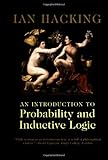# ネイマン自身の信頼区間の解釈とフィッシャーの立ち位置のメモ

ネイマン自身の信頼区間の解釈とフィッシャーの立場について、Ian Hackingの「An introduction to probability and inductive logic」から引用のメモをしておきます。

ネイマン自身の信頼区間の解釈について(p242)：

Neyman said that when we use the method of confidence intervals, we are not making inductive inferences. Instead, we are practicing inductive behavior. If we use confidence intervals, our behavior will have the desired result 95% of the time. If we use 99% intervals, our behavior will have the desired result 99% of the time.

フィッシャーのベイジアンおよびネイマン-ピアソン派に対する立ち位置について(p226)：

Fisher thought Bayesian reasoning was fine in its place, when one had good scientific ground for a prior probability. He thought that the Neyman-Pearson framework was fine for quality control, but not for determining single, important scientific quantities. Above all, he thought that statistics and frequency-type probabilities were an essential tool for the communication of information among scientists working in fields where there may not be very good theories about nature, but where there is a great deal of data which needs to be understood.An Introduction to Probability and Inductive Logic